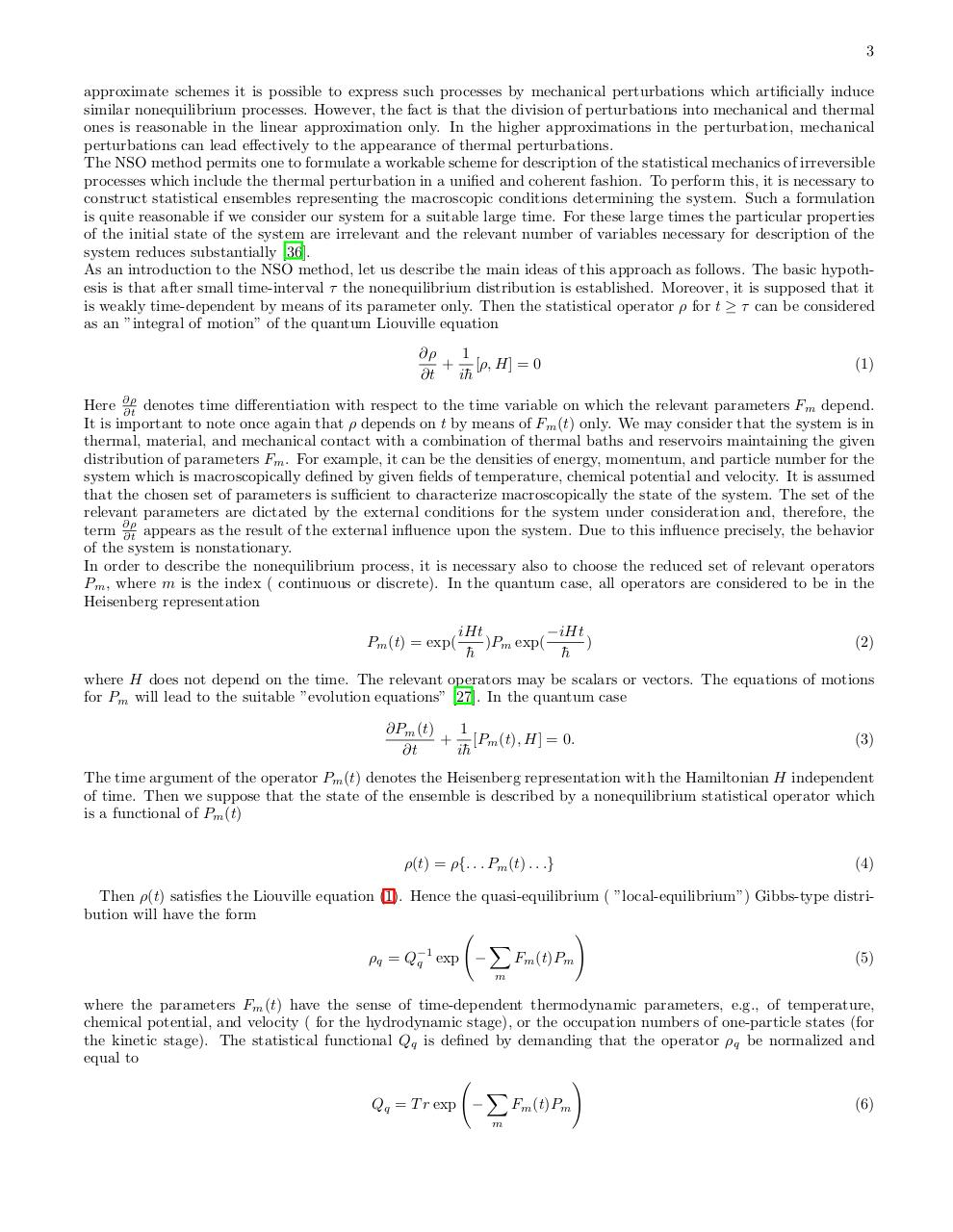# PDF Archive

Easily share your PDF documents with your contacts, on the Web and Social Networks.

## Nonequilibrium Statistical Operator IJMPB05.pdfPage 1 2 34521

#### Text preview

3
approximate schemes it is possible to express such processes by mechanical perturbations which artificially induce
similar nonequilibrium processes. However, the fact is that the division of perturbations into mechanical and thermal
ones is reasonable in the linear approximation only. In the higher approximations in the perturbation, mechanical
perturbations can lead effectively to the appearance of thermal perturbations.
The NSO method permits one to formulate a workable scheme for description of the statistical mechanics of irreversible
processes which include the thermal perturbation in a unified and coherent fashion. To perform this, it is necessary to
construct statistical ensembles representing the macroscopic conditions determining the system. Such a formulation
is quite reasonable if we consider our system for a suitable large time. For these large times the particular properties
of the initial state of the system are irrelevant and the relevant number of variables necessary for description of the
system reduces substantially .
As an introduction to the NSO method, let us describe the main ideas of this approach as follows. The basic hypothesis is that after small time-interval τ the nonequilibrium distribution is established. Moreover, it is supposed that it
is weakly time-dependent by means of its parameter only. Then the statistical operator ρ for t ≥ τ can be considered
as an ”integral of motion” of the quantum Liouville equation
∂ρ
1
+ [ρ, H] = 0
∂t
i¯h

(1)

Here ∂ρ
∂t denotes time differentiation with respect to the time variable on which the relevant parameters Fm depend.
It is important to note once again that ρ depends on t by means of Fm (t) only. We may consider that the system is in
thermal, material, and mechanical contact with a combination of thermal baths and reservoirs maintaining the given
distribution of parameters Fm . For example, it can be the densities of energy, momentum, and particle number for the
system which is macroscopically defined by given fields of temperature, chemical potential and velocity. It is assumed
that the chosen set of parameters is sufficient to characterize macroscopically the state of the system. The set of the
relevant parameters are dictated by the external conditions for the system under consideration and, therefore, the
term ∂ρ
∂t appears as the result of the external influence upon the system. Due to this influence precisely, the behavior
of the system is nonstationary.
In order to describe the nonequilibrium process, it is necessary also to choose the reduced set of relevant operators
Pm , where m is the index ( continuous or discrete). In the quantum case, all operators are considered to be in the
Heisenberg representation
Pm (t) = exp(

iHt
−iHt
)Pm exp(
)
¯h
¯h

(2)

where H does not depend on the time. The relevant operators may be scalars or vectors. The equations of motions
for Pm will lead to the suitable ”evolution equations” . In the quantum case
1
∂Pm (t)
+ [Pm (t), H] = 0.
∂t
i¯h

(3)

The time argument of the operator Pm (t) denotes the Heisenberg representation with the Hamiltonian H independent
of time. Then we suppose that the state of the ensemble is described by a nonequilibrium statistical operator which
is a functional of Pm (t)
ρ(t) = ρ{. . . Pm (t) . . .}

(4)

Then ρ(t) satisfies the Liouville equation (1). Hence the quasi-equilibrium ( ”local-equilibrium”) Gibbs-type distribution will have the form
!
X
−1
(5)
ρq = Qq exp −
Fm (t)Pm
m

where the parameters Fm (t) have the sense of time-dependent thermodynamic parameters, e.g., of temperature,
chemical potential, and velocity ( for the hydrodynamic stage), or the occupation numbers of one-particle states (for
the kinetic stage). The statistical functional Qq is defined by demanding that the operator ρq be normalized and
equal to
!
X
(6)
Qq = T r exp −
Fm (t)Pm
m